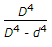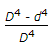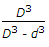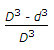# Civil Engineering - UPSC Civil Service Exam Questions

6.

The axial load which just produces the condition of elastic instability in a column is

Explanation:

No answer description available for this question. Let us discuss.

7.

If R is the radius of the circular railway curve in metres and D is the degree of the curve, then which one of the following is the expression for D ?

 A. D = 360/R B. D = 1080/R C. D = 1720/R D. D = 720/R

Explanation:

No answer description available for this question. Let us discuss.

8.

Given below are methods of compaction :
1. Vibration technique
2. Flooding the soil
3. Sheeps-foot roller
4. Tandem roller
5. Heavy weights dropped from a height.
The methods suitable for cohesionless soils include

 A. 1, 2 and 3 B. 2, 3 and 4 C. 1, 2 and 5 D. 3, 4 and 5

Explanation:

No answer description available for this question. Let us discuss.

9.

The ratio of torsional moments of resistance of a solid circular shaft of diameter D and a hollow shaft having external diameter D and internal diameter d is given by

 A.B.C.D.Explanation:

No answer description available for this question. Let us discuss.

10.

Consider the following statements relating to triaxial tests :
1. Change in length of the specimen in a triaxial shear test is required to be accounted for in the calculation of stresses at failure.
2. In 'Drained Triaxial Shear Test', drainage is permitted under a specified all-round pressure until consolidation is complete. The principal stress difference is then applied with no drainage being permitted.
3. Unconfined compression test is a special case of triaxial shear test.
Of these statements :

 A. 1 and 2 are correct B. 1 and 3 are correct C. 2 and 3 are correct D. 1, 2 and 3 are correct